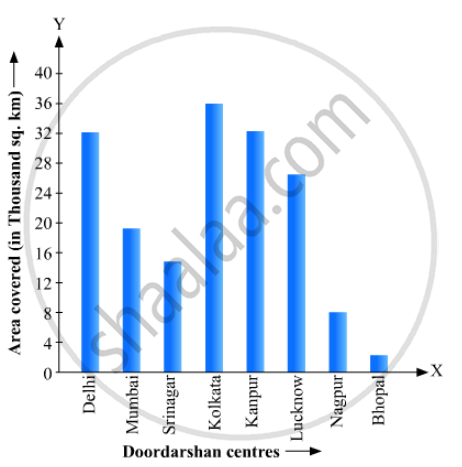# Read the Bar Graph Given in Fig. 23.22 and Answer the Following Questions:(I) What Information is Given by the Bar Graph? (Ii) Which Doordarshan Centre Covers Maximum Area? Also Tell the Covered Area. - Mathematics

Read the bar graph given in Fig. 23.22 and answer the following questions:(i) What information is given by the bar graph?
(ii) Which Doordarshan centre covers maximum area? Also tell the covered area.
(iii) What is the difference between the areas covered by the centres at delhi and Bombay?
(iv) Which Doordarshan centres are in U.P State? What are the areas covered by them?

#### Solution

(1) The bar graph represents the area of coverage (in 1000 square km) of some Doordarshan Centers of India.

(2) It is seen from the bar graph that the height of the 4th bar from the left is maximum, which is corresponding to Kolkata. Hence, the Kolkata Doordarshan covers maximum area. The area covered by Kolkata Doordarshan is

36 ×1000

= 36000 sq.km

(3) The area covered by Delhi Doordarshan is

34 ×1000

= 34000 sq.km

The area covered by Mumbai Doordarshan is

20 ×1000

= 20000 sq.km

Their difference is 34000 – 20000 = 14000 sq. km.

(4) The Doordarshan centers in Kanpur and Lucknow are in the U.P. state. The area covered by Kanpur Doordarshan is

32 ×1000

= 32000 sq.km

The area covered by Lucknow Doordarshan is

25 ×1000

= 25000 sq.km

Concept: Graphical Representation of Data
Is there an error in this question or solution?

#### APPEARS IN

RD Sharma Mathematics for Class 9
Chapter 23 Graphical Representation of Statistical Data
Exercise 23.1 | Q 18 | Page 17

Share